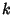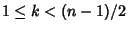## Pósa's Theorem

Letbe a Simple Graph withVertices.

1. If, for everyin, the number of Vertices of Valency not exceedingis less than, and

2. If, forOdd, the number of Vertices with Valency not exceedingis less than or equal to,
thencontains a Hamiltonian Circuit.